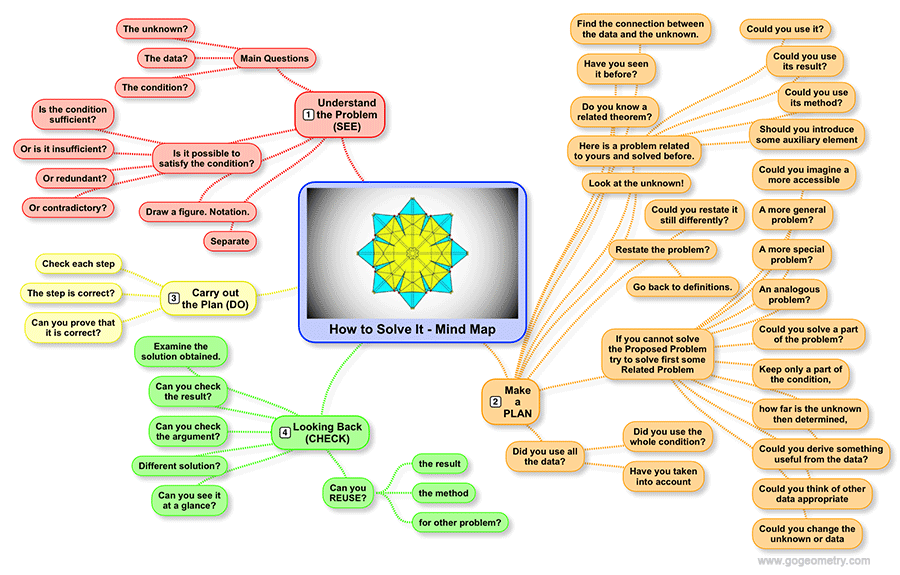# Geometry Problem 1252

### Elements: Triangle, Circle, Circumcircle, Altitude, Area, Hexagon, Perpendicular, 90 Degrees. Mind Map.

In a triangle ABC, the altitudes from A, B, and C meet the circumcircle O at D, E, and F, respectively as shown in the figure below. Prove that the area of triangle ABC is equal to one-half the area of the hexagon AFBDCE.## "How to Solve It" Mind Map

George Polya's 1945 book "How to Solve It, A new aspect of Mathematical Method", is a book describing methods of problem solving. It suggests the following steps when solving a mathematical problem: (1) First, Understand the problem. (2) Make a plan. (3) Carry out the plan. (4) Look back on your solution.